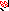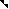# MINLPLib

### A Library of Mixed-Integer and Continuous Nonlinear Programming Instances

#### Instance pointpack04

`Find the maximum radius of 4 non-overlapping circles that all lie in the unix-box.`
 Formatsⓘ ams gms lp mod nl osil pip py Primal Bounds (infeas ≤ 1e-08)ⓘ 0.00000000 p1 ( gdx sol ) (infeas: 0)1.00000000 p2 ( gdx sol ) (infeas: 0) Other points (infeas > 1e-08)ⓘ Dual Boundsⓘ 1.00000000 (ANTIGONE)1.00000000 (BARON)1.00000000 (COUENNE)1.00000000 (GUROBI)1.00000000 (LINDO)1.00000005 (SCIP) Referencesⓘ Anstreicher, Kurt, Semidefinite programming versus the reformulation-linearization technique for nonconvex quadratically constrained quadratic programming, Journal of Global Optimization, 43:2, 2009, 471-484. Sourceⓘ ANTIGONE test library model Other_MIQCQP/pnt_pack_04.gms Applicationⓘ Geometry Added to libraryⓘ 15 Aug 2014 Problem typeⓘ QCP #Variablesⓘ 9 #Binary Variablesⓘ 0 #Integer Variablesⓘ 0 #Nonlinear Variablesⓘ 8 #Nonlinear Binary Variablesⓘ 0 #Nonlinear Integer Variablesⓘ 0 Objective Senseⓘ max Objective typeⓘ linear Objective curvatureⓘ linear #Nonzeros in Objectiveⓘ 1 #Nonlinear Nonzeros in Objectiveⓘ 0 #Constraintsⓘ 10 #Linear Constraintsⓘ 4 #Quadratic Constraintsⓘ 6 #Polynomial Constraintsⓘ 0 #Signomial Constraintsⓘ 0 #General Nonlinear Constraintsⓘ 0 Operands in Gen. Nonlin. Functionsⓘ Constraints curvatureⓘ concave #Nonzeros in Jacobianⓘ 38 #Nonlinear Nonzeros in Jacobianⓘ 24 #Nonzeros in (Upper-Left) Hessian of Lagrangianⓘ 32 #Nonzeros in Diagonal of Hessian of Lagrangianⓘ 8 #Blocks in Hessian of Lagrangianⓘ 2 Minimal blocksize in Hessian of Lagrangianⓘ 4 Maximal blocksize in Hessian of Lagrangianⓘ 4 Average blocksize in Hessian of Lagrangianⓘ 4.0 #Semicontinuitiesⓘ 0 #Nonlinear Semicontinuitiesⓘ 0 #SOS type 1ⓘ 0 #SOS type 2ⓘ 0 Minimal coefficientⓘ 1.0000e+00 Maximal coefficientⓘ 2.0000e+00 Infeasibility of initial pointⓘ 0 Sparsity JacobianⓘSparsity Hessian of Lagrangianⓘ```\$offlisting
*
*  Equation counts
*      Total        E        G        L        N        X        C        B
*         10        0        0       10        0        0        0        0
*
*  Variable counts
*                   x        b        i      s1s      s2s       sc       si
*      Total     cont   binary  integer     sos1     sos2    scont     sint
*          9        9        0        0        0        0        0        0
*  FX      0
*
*  Nonzero counts
*      Total    const       NL      DLL
*         38       14       24        0
*
*  Solve m using NLP maximizing objvar;

Variables  x1,x2,x3,x4,x5,x6,x7,x8,objvar;

Positive Variables  x3,x4,x5,x6,x7,x8;

Equations  e1,e2,e3,e4,e5,e6,e7,e8,e9,e10;

e1.. 2*x1*x2 - x1*x1 - x2*x2 - x5*x5 + 2*x5*x6 - x6*x6 + objvar =L= 0;

e2.. 2*x1*x3 - x1*x1 - x3*x3 - x5*x5 + 2*x5*x7 - x7*x7 + objvar =L= 0;

e3.. 2*x1*x4 - x1*x1 - x4*x4 - x5*x5 + 2*x5*x8 - x8*x8 + objvar =L= 0;

e4.. 2*x2*x3 - x2*x2 - x3*x3 - x6*x6 + 2*x6*x7 - x7*x7 + objvar =L= 0;

e5.. 2*x2*x4 - x2*x2 - x4*x4 - x6*x6 + 2*x6*x8 - x8*x8 + objvar =L= 0;

e6.. 2*x3*x4 - x3*x3 - x4*x4 - x7*x7 + 2*x7*x8 - x8*x8 + objvar =L= 0;

e7..  - x5 + x6 =L= 0;

e8..  - x1 + x2 =L= 0;

e9..  - x2 + x3 =L= 0;

e10..  - x3 + x4 =L= 0;

* set non-default bounds
x1.lo = 0.5; x1.up = 1;
x2.lo = 0.5; x2.up = 1;
x3.up = 1;
x4.up = 1;
x5.up = 1;
x6.up = 1;
x7.up = 1;
x8.up = 1;

Model m / all /;

m.limrow=0; m.limcol=0;
m.tolproj=0.0;

\$if NOT '%gams.u1%' == '' \$include '%gams.u1%'

\$if not set NLP \$set NLP NLP
Solve m using %NLP% maximizing objvar;

```

Last updated: 2023-03-21 Git hash: 0a21635c